# Hyperelastic material model Arruda-Boyce for Nonlinear Finite Element Analysis

March 15, 2021

In structural finite element analysis, non-metallic materials such as rubber and biological materials are often encountered. Because the mechanical properties of these materials are very different from those of metal materials, such as elasticity, large deformation, incompressibility, and so on. Scientists and researchers have grouped this type of material into one category and call them hyperelastic material(model).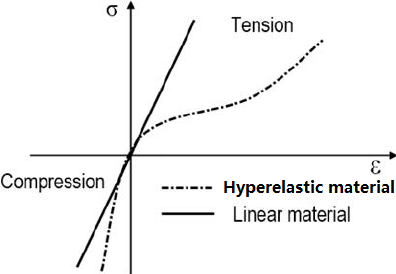These hyperelastic models or materials have significant features:

1. Can sustain large elastic (recoverable) deformation, the strain can reach 100–800%;
2. Since the deformation is related to the straightening of the molecular chain of the material, the volume change is infinitesimal. Therefore, hyperelastic materials are almost incompressible;
3. The stress-strain relationship exhibits strong nonlinearity; usually, under tension, the material softens and then hardens, and the material hardens sharply when it is compressed.

Common hyperelastic models are Neo-Hookean, Mooney-Rivlin, Odgen, Arruda-Boyce, Gent, Yeoh, Blatz-Ko, etc. WELSIM supports these hyperelastic models. In this article, we only focus on the Arruda-Boyce model.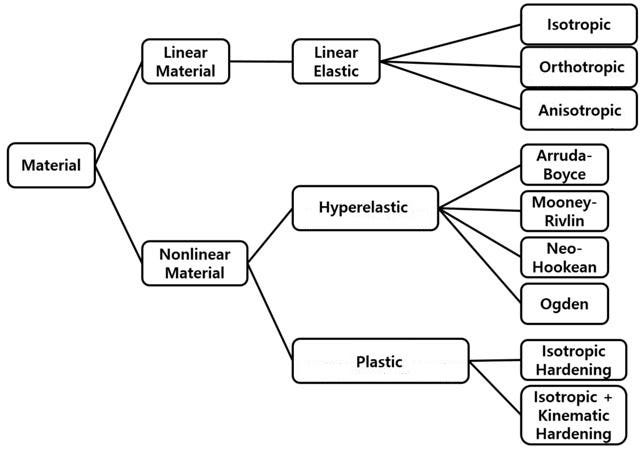In 1993, Professor Boyce of MIT and her doctoral student Arruda published an article called “A three-dimensional constitutive model for the large stretch behavior of rubber elastic materials” on the Journal of the Mechanics and Physics of Solids, one of the most well-known journals in the field of mechanics. This paper builds the foundation of the Arruda-Boyce model.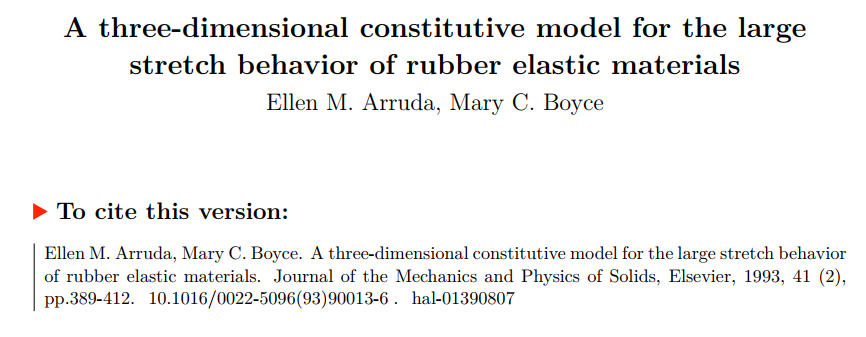It can be seen that the Arruda-Boyce model is relatively new compared to those hyperelastic models from the 50s to 60s. And from the title of the article, we can tell that the Arruda-Boyce model has strengths in modeling the hyperelastic material that is subjected to large stretches.

People who are familiar with the hyperelastic model know that we always use the elastic strain energy(potential) to describe hyperelastic characteristics. The function of Arruda-Boyce’s elastic strain energy is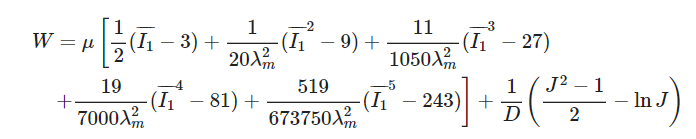where

• u is the initial shear modulus of the material.
• lambda is a limiting network stretch.
• D is the material incompressible parameter. When the term containing D is zero, the material combines completely incompressible.
• J is the Jacobin for volume changes. Mathematically it is the determinant of the deformation gradient.
• I_1 is the first invariant of the Cauchy-Green tensor.

Arruda-Boyce model features can be summarized as follows:

• The default is an incompressible hyperelastic model. Compressible characteristics are introduced by adding that items containing volume change term J.
• When the limiting network stretch becomes infinite, the Arruda-Boyce model is equivalent to the Neo-Hookean model.
• The limiting network stretch must be greater than or equal to 1.
• Incompressible parameter D1 cannot be zero.
• The Arruda-Boyce model evolved from the molecular structure of hyperelastic materials and adopted the 8-chain molecular structure as a prototype. The model performs well in both uniaxial and biaxial stretching.

In general, the Arruda-Boyce model is easy-of-use and requires only three input parameters. It has accuracy advantages in high stretch conditions. These parameter values need to be determined according to the experimental data or literature references. These parameters can also be determined by the curve fitting numerical method of the test data.

### Examples of nonlinear hyperelastic materials analysis

In this section, we use the finite element software WELSIM to simulate a rubber material test piece that is under tensile stretch. The full-size model is used, with no symmetry assumption. We constrain one end of the working piece and impose a displacement boundary condition on another end. The stress and reaction force results are computed and verified.

Analysis procedure:

(1) Set the unit system to U.S.Customary (inch) and create a structural static analysis project.

(2) Set material properties.

Create a new material object. Double-click this material object to enter edit mode. From the hyperelastic material properties tab, toggle Arruda Boyce property. And assign values: Mu = 1.33e5 Pa, lambda = 1, D1 = 4.3e-6 1 / Pa. When parameters are input, the stress-strain curves of the corresponding settings display in the graph window. Change the name of the material object to ArrudaBoyceMat.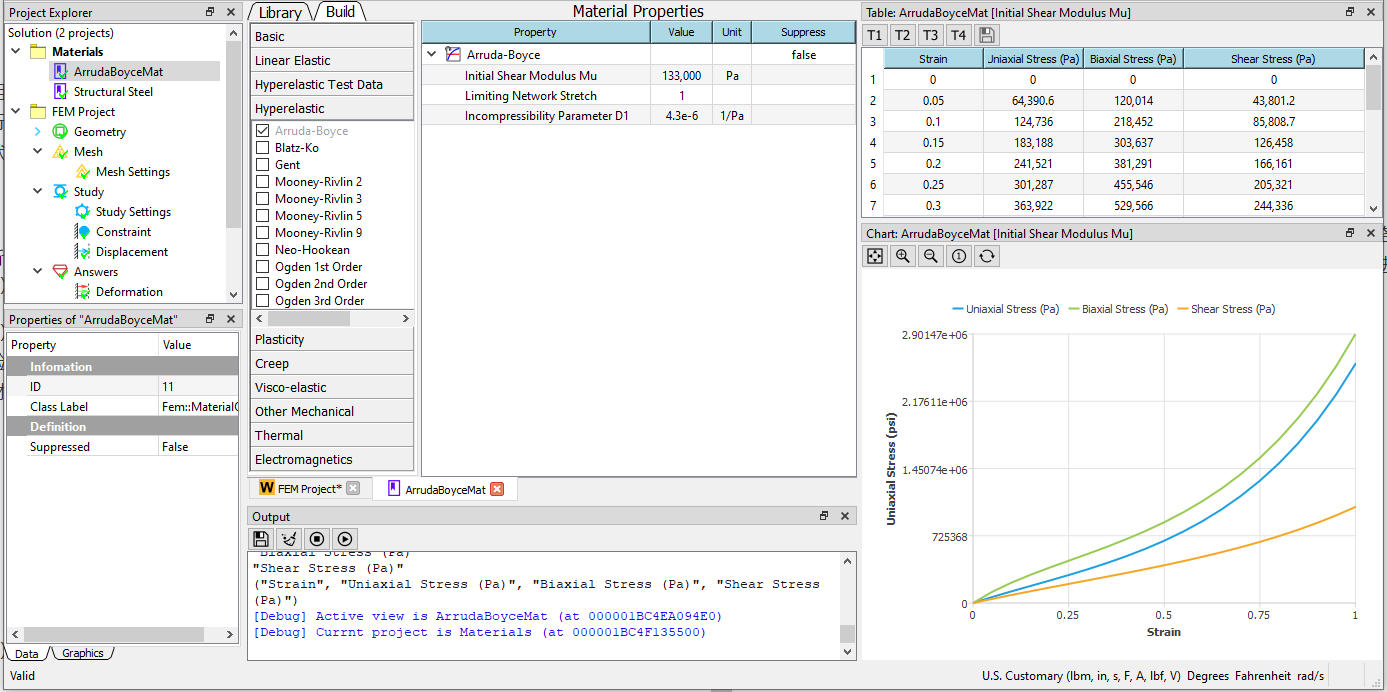(3) Create geometry

Add a new Box and set the size to 1x1x10 inches. Assign the ArrudaBoyceMat material defined in the previous step to this body.

(4) Mesh generation Set the maximum cell size to 0.3 inches and use a higher-order element. After meshing, 4037 nodes and 2240 Tet10 units were generated.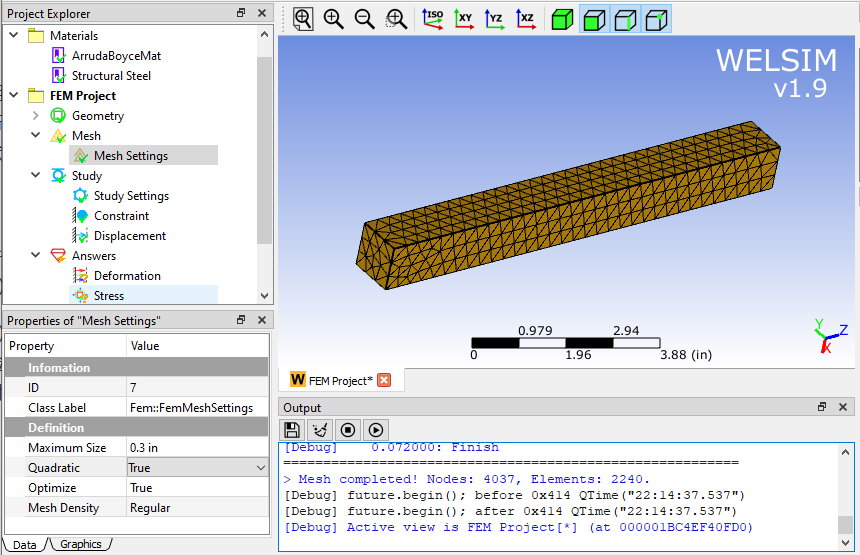(5) Constraints and loads Apply constraints on the left end. To demonstrate the advantages of Arruda-Boyce under large stretch, a 3-inch displacement in the Z direction is set on the right end of the body.

(6) Study settings, solve, and post-processing of results. Since the material is nonlinear, we set 10 substeps to ease the convergence. Click the solve button to start the calculation.

Evaluate Von-Mises stress.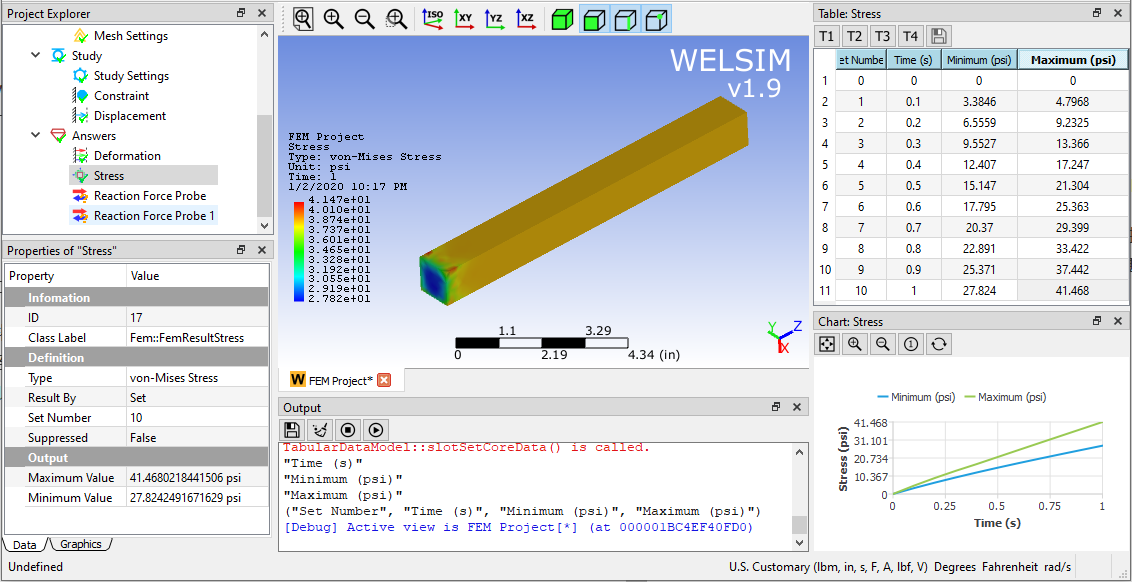Evaluate the reaction force at the fixed end. The reaction force results match the stress results.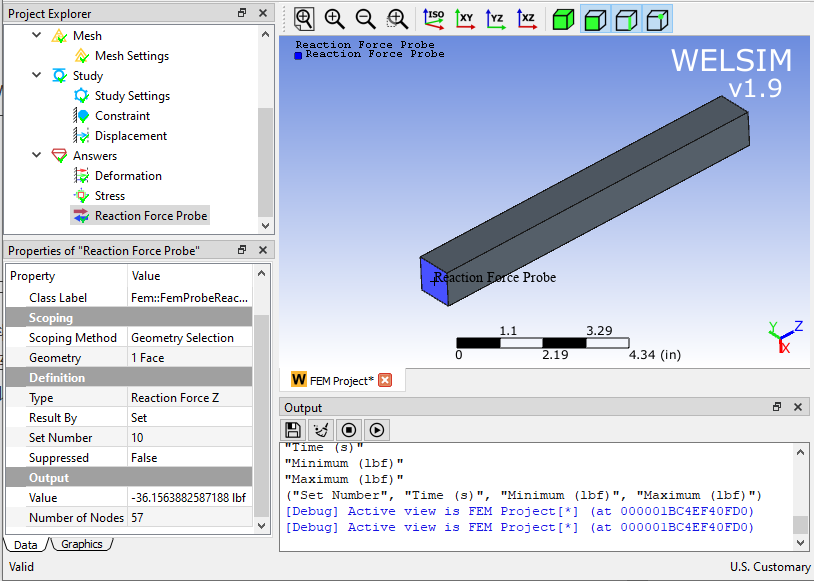Before the application of finite element software, the calculation and prediction of nonlinear materials were complicated. Manually calculating the deformation, stress, and reaction force of hyperelastic materials often took a lot of time and work. Now with the finite element software, mechanical analysis becomes faster, more accurate and more fun.